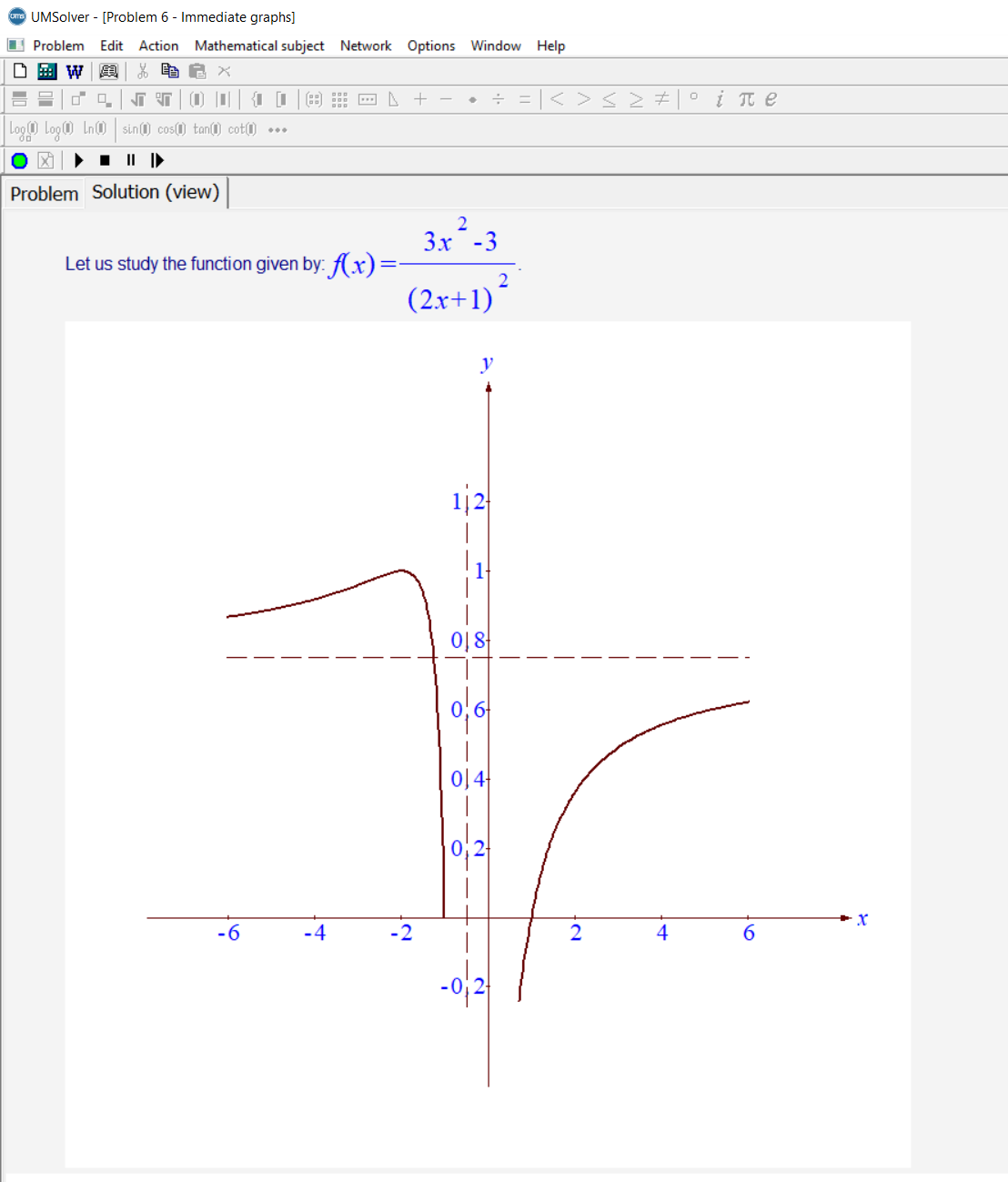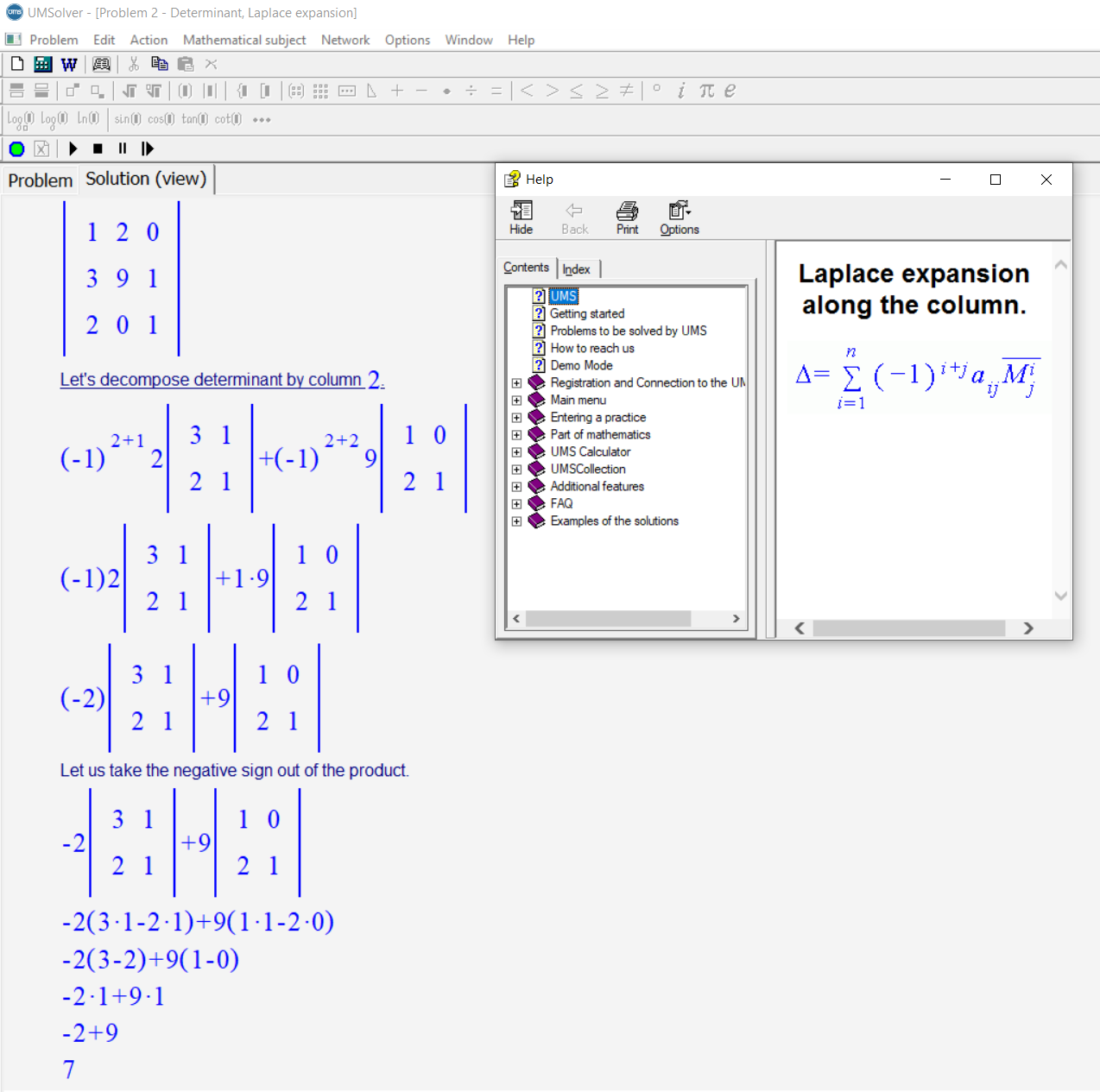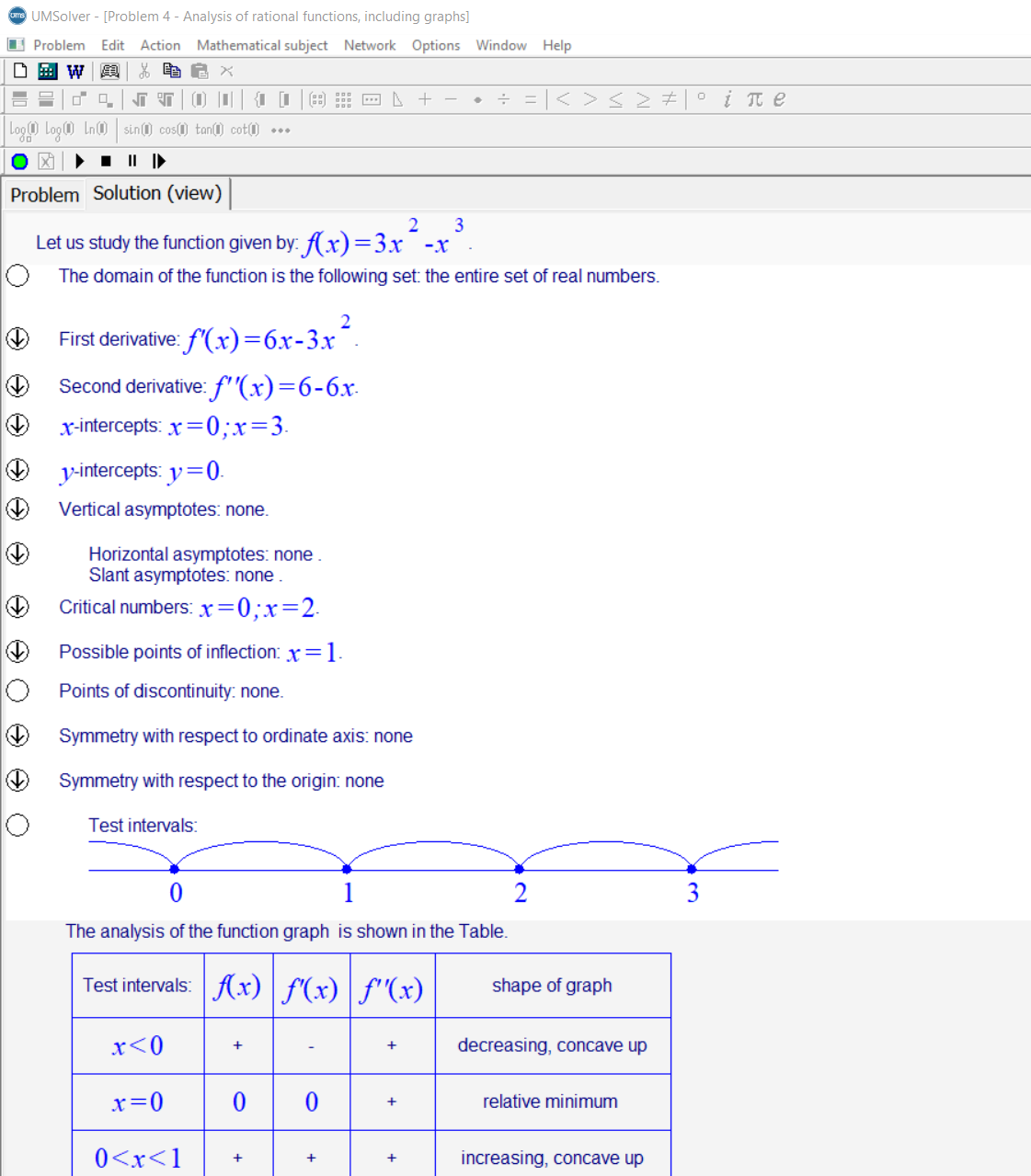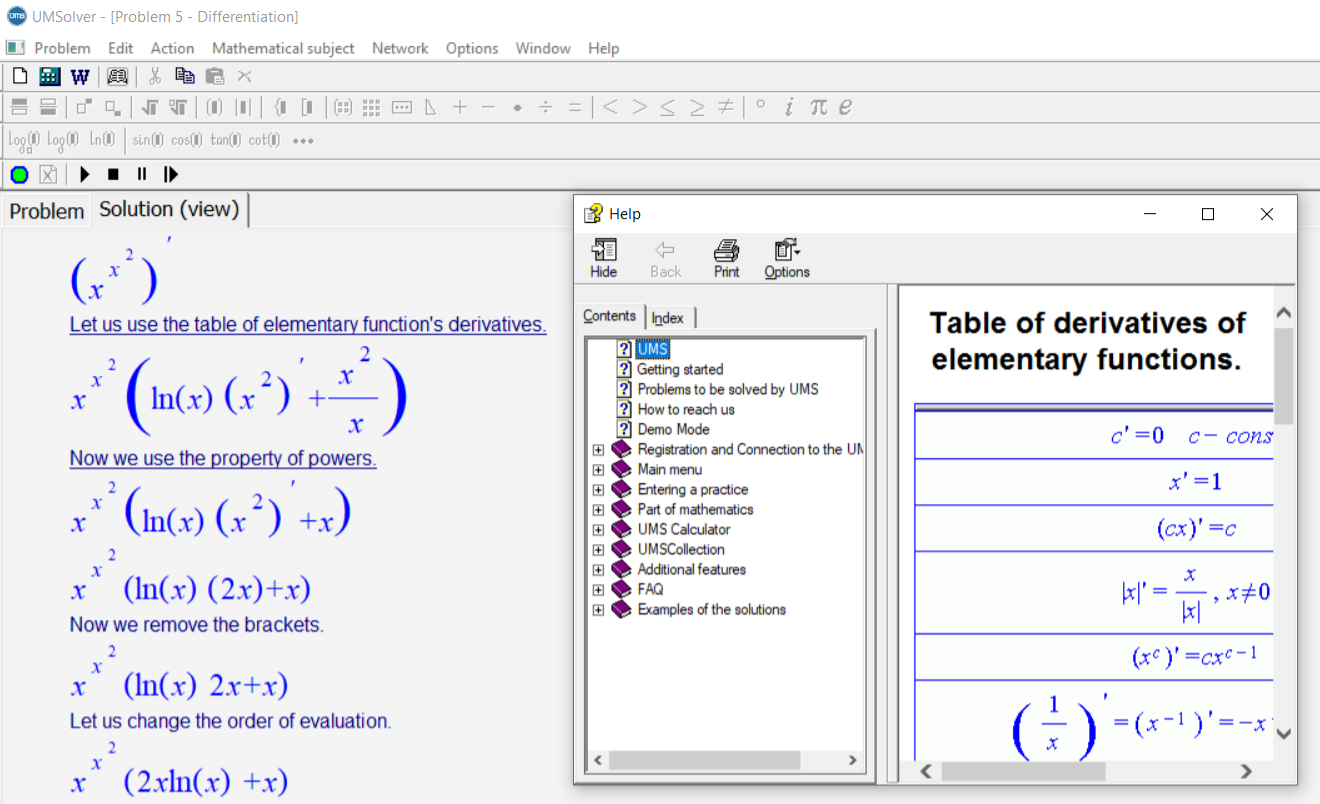# For College Students

Topics for Advanced Placement and University students

### Universal Math Solver has the following modules for college and university students and advanced placement math classes:

• Matrices and Determinants (algebraic operations, the inverse of a matrix, the determinant, rank of a matrix)
• Run a complete analysis of rational functions with derivatives
• Differentiation (partial differentiation) of any functions
• Graphing of any functions### In the module “Matrices and Determinants” you can find:

• Linear Combination of several Matrices of the same size
• Product of two Matrices
• A Square Matrix to the n-th (natural number) Power
• Determinant of the Matrix using Gauss algorithm
• Determinant of the Matrix using Laplace expansion
• Determinant of the Matrix using Sarrus’ rule
• Rank of Matrix
• Inverse of Matrix using Determinant
• Inverse of Matrix using Gauss-Jordan Method

### Complete analysis of rational functions includes:

• Domain and Range of functions
• x-intercepts and y-intercepts
• Symmetry
• Points of discontinuity
• Vertical asymptotes
• Horizontal asymptotes
• Relative extrema
• Concavity
• Points of inflection### The distinctive features of UMS software in contrast to other software:

• Complete step by step explanation of the analysis of the function.
• Output of the complete table of the results of the analysis (exactly as provided in Calculus courses).
• Automatic scaling of plot, so that all the critical plotting points and aspects of the graph are demonstrated.
• Complete demonstration of critical aspects of the function (extrema, asymptotes, inflection points, etc.)

For Windows OS